# 12.5 Conic sections in polar coordinates  (Page 5/8)

 Page 5 / 8

For the following exercises, convert the polar equation of a conic section to a rectangular equation.

$25{x}^{2}+16{y}^{2}-12y-4=0$

$21{x}^{2}-4{y}^{2}-30x+9=0$

$64{y}^{2}=48x+9$

$96{y}^{2}-25{x}^{2}+110y+25=0$

$3{x}^{2}+4{y}^{2}-2x-1=0$

$5{x}^{2}+9{y}^{2}-24x-36=0$

For the following exercises, graph the given conic section. If it is a parabola, label the vertex, focus, and directrix. If it is an ellipse, label the vertices and foci. If it is a hyperbola, label the vertices and foci.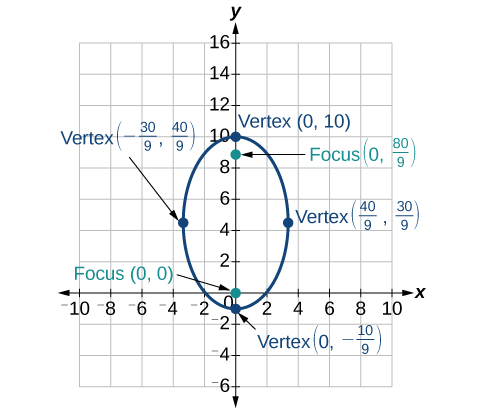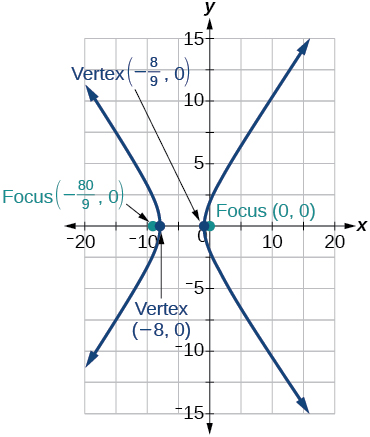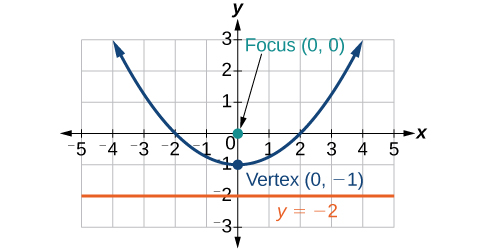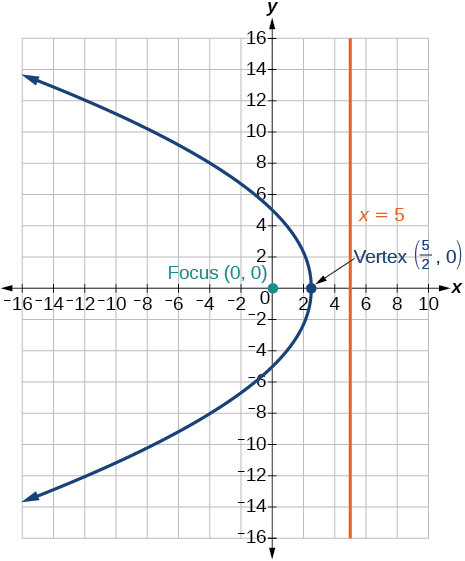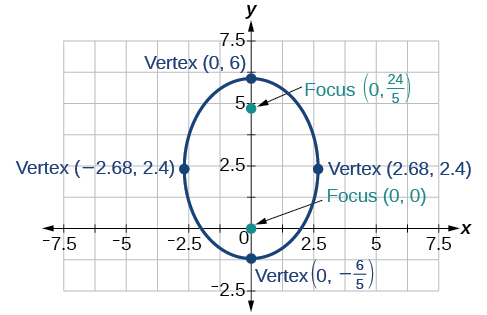For the following exercises, find the polar equation of the conic with focus at the origin and the given eccentricity and directrix.

Directrix: $x=4;\text{\hspace{0.17em}}e=\frac{1}{5}$

$r=\frac{4}{5+\mathrm{cos}\theta }$

Directrix: $x=-4;\text{\hspace{0.17em}}e=5$

Directrix: $y=2;\text{\hspace{0.17em}}e=2$

$r=\frac{4}{1+2\mathrm{sin}\theta }$

Directrix: $y=-2;\text{\hspace{0.17em}}e=\frac{1}{2}$

Directrix: $x=1;\text{\hspace{0.17em}}e=1$

$r=\frac{1}{1+\mathrm{cos}\theta }$

Directrix: $x=-1;\text{\hspace{0.17em}}e=1$

Directrix: $x=-\frac{1}{4};\text{\hspace{0.17em}}e=\frac{7}{2}$

$r=\frac{7}{8-28\mathrm{cos}\theta }$

Directrix: $y=\frac{2}{5};\text{\hspace{0.17em}}e=\frac{7}{2}$

Directrix: $y=4;\text{\hspace{0.17em}}e=\frac{3}{2}$

$r=\frac{12}{2+3\mathrm{sin}\theta }$

Directrix: $x=-2;\text{\hspace{0.17em}}e=\frac{8}{3}$

Directrix: $x=-5;\text{\hspace{0.17em}}e=\frac{3}{4}$

$r=\frac{15}{4-3\mathrm{cos}\theta }$

Directrix: $y=2;\text{\hspace{0.17em}}e=2.5$

Directrix: $x=-3;\text{\hspace{0.17em}}e=\frac{1}{3}$

$r=\frac{3}{3-3\mathrm{cos}\theta }$

## Extensions

Recall from Rotation of Axes that equations of conics with an $\text{\hspace{0.17em}}xy\text{\hspace{0.17em}}$ term have rotated graphs. For the following exercises, express each equation in polar form with $\text{\hspace{0.17em}}r\text{\hspace{0.17em}}$ as a function of $\text{\hspace{0.17em}}\theta .$

$xy=2$

${x}^{2}+xy+{y}^{2}=4$

$r=±\frac{2}{\sqrt{1+\mathrm{sin}\theta \mathrm{cos}\theta }}$

$2{x}^{2}+4xy+2{y}^{2}=9$

$16{x}^{2}+24xy+9{y}^{2}=4$

$r=±\frac{2}{4\mathrm{cos}\theta +3\mathrm{sin}\theta }$

$2xy+y=1$

## The Ellipse

For the following exercises, write the equation of the ellipse in standard form. Then identify the center, vertices, and foci.

$\frac{{x}^{2}}{25}+\frac{{y}^{2}}{64}=1$

$\frac{{x}^{2}}{{5}^{2}}+\frac{{y}^{2}}{{8}^{2}}=1;\text{\hspace{0.17em}}$ center: $\text{\hspace{0.17em}}\left(0,0\right);\text{\hspace{0.17em}}$ vertices: $\text{\hspace{0.17em}}\left(5,0\right),\left(-5,0\right),\left(0,8\right),\left(0,-8\right);\text{\hspace{0.17em}}$ foci: $\text{\hspace{0.17em}}\left(0,\sqrt{39}\right),\left(0,-\sqrt{39}\right)$

$\frac{{\left(x-2\right)}^{2}}{100}+\frac{{\left(y+3\right)}^{2}}{36}=1$

$9{x}^{2}+{y}^{2}+54x-4y+76=0$

$\frac{{\left(x+3\right)}^{2}}{{1}^{2}}+\frac{{\left(y-2\right)}^{2}}{{3}^{2}}=1\text{\hspace{0.17em}}\text{\hspace{0.17em}}\left(-3,2\right);\text{\hspace{0.17em}}\text{\hspace{0.17em}}\left(-2,2\right),\left(-4,2\right),\left(-3,5\right),\left(-3,-1\right);\text{\hspace{0.17em}}\text{\hspace{0.17em}}\left(-3,2+2\sqrt{2}\right),\left(-3,2-2\sqrt{2}\right)$

$9{x}^{2}+36{y}^{2}-36x+72y+36=0$

For the following exercises, graph the ellipse, noting center, vertices, and foci.

$\frac{{x}^{2}}{36}+\frac{{y}^{2}}{9}=1$

center: $\text{\hspace{0.17em}}\left(0,0\right);\text{\hspace{0.17em}}$ vertices: $\text{\hspace{0.17em}}\left(6,0\right),\left(-6,0\right),\left(0,3\right),\left(0,-3\right);\text{\hspace{0.17em}}$ foci: $\text{\hspace{0.17em}}\left(3\sqrt{3},0\right),\left(-3\sqrt{3},0\right)$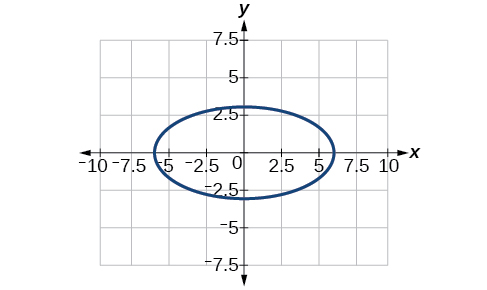$\frac{{\left(x-4\right)}^{2}}{25}+\frac{{\left(y+3\right)}^{2}}{49}=1$

$4{x}^{2}+{y}^{2}+16x+4y-44=0$

center: $\text{\hspace{0.17em}}\left(-2,-2\right);\text{\hspace{0.17em}}$ vertices: $\text{\hspace{0.17em}}\left(2,-2\right),\left(-6,-2\right),\left(-2,6\right),\left(-2,-10\right);\text{\hspace{0.17em}}$ foci: $\text{\hspace{0.17em}}\left(-2,-2+4\sqrt{3},\right),\left(-2,-2-4\sqrt{3}\right)$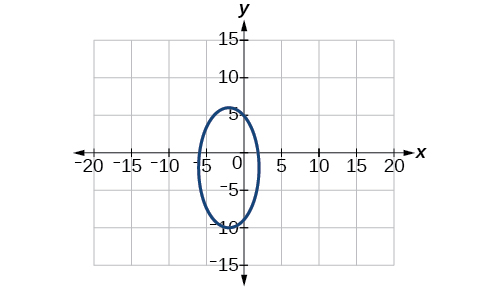$\text{\hspace{0.17em}}2{x}^{2}+3{y}^{2}-20x+12y+38=0$

For the following exercises, use the given information to find the equation for the ellipse.

Center at $\text{\hspace{0.17em}}\left(0,0\right),$ focus at $\text{\hspace{0.17em}}\left(3,0\right),$ vertex at $\text{\hspace{0.17em}}\left(-5,0\right)$

$\frac{{x}^{2}}{25}+\frac{{y}^{2}}{16}=1$

Center at $\text{\hspace{0.17em}}\left(2,-2\right),$ vertex at $\text{\hspace{0.17em}}\left(7,-2\right),$ focus at $\text{\hspace{0.17em}}\left(4,-2\right)$

A whispering gallery is to be constructed such that the foci are located 35 feet from the center. If the length of the gallery is to be 100 feet, what should the height of the ceiling be?

Approximately 35.71 feet

## The Hyperbola

For the following exercises, write the equation of the hyperbola in standard form. Then give the center, vertices, and foci.

$\frac{{x}^{2}}{81}-\frac{{y}^{2}}{9}=1$

$\frac{{\left(y+1\right)}^{2}}{16}-\frac{{\left(x-4\right)}^{2}}{36}=1$

$\frac{{\left(y+1\right)}^{2}}{{4}^{2}}-\frac{{\left(x-4\right)}^{2}}{{6}^{2}}=1;\text{\hspace{0.17em}}$ center: $\text{\hspace{0.17em}}\left(4,-1\right);\text{\hspace{0.17em}}$ vertices: $\text{\hspace{0.17em}}\left(4,3\right),\left(4,-5\right);\text{\hspace{0.17em}}$ foci: $\text{\hspace{0.17em}}\left(4,-1+2\sqrt{13}\right),\left(4,-1-2\sqrt{13}\right)$

$9{y}^{2}-4{x}^{2}+54y-16x+29=0$

$3{x}^{2}-{y}^{2}-12x-6y-9=0$

$\frac{{\left(x-2\right)}^{2}}{{2}^{2}}-\frac{{\left(y+3\right)}^{2}}{{\left(2\sqrt{3}\right)}^{2}}=1;\text{\hspace{0.17em}}$ center: $\text{\hspace{0.17em}}\left(2,-3\right);\text{\hspace{0.17em}}$ vertices: $\text{\hspace{0.17em}}\left(4,-3\right),\left(0,-3\right);\text{\hspace{0.17em}}$ foci: $\text{\hspace{0.17em}}\left(6,-3\right),\left(-2,-3\right)$

For the following exercises, graph the hyperbola, labeling vertices and foci.

#### Questions & Answers

write down the polynomial function with root 1/3,2,-3 with solution
if A and B are subspaces of V prove that (A+B)/B=A/(A-B)
write down the value of each of the following in surd form a)cos(-65°) b)sin(-180°)c)tan(225°)d)tan(135°)
Prove that (sinA/1-cosA - 1-cosA/sinA) (cosA/1-sinA - 1-sinA/cosA) = 4
what is the answer to dividing negative index
In a triangle ABC prove that. (b+c)cosA+(c+a)cosB+(a+b)cisC=a+b+c.
give me the waec 2019 questions
the polar co-ordinate of the point (-1, -1)
prove the identites sin x ( 1+ tan x )+ cos x ( 1+ cot x )= sec x + cosec x
tanh`(x-iy) =A+iB, find A and B
B=Ai-itan(hx-hiy)
Rukmini
Give me the reciprocal of even number
Aliyu
what is the addition of 101011 with 101010
If those numbers are binary, it's 1010101. If they are base 10, it's 202021.
Jack
extra power 4 minus 5 x cube + 7 x square minus 5 x + 1 equal to zero
the gradient function of a curve is 2x+4 and the curve passes through point (1,4) find the equation of the curve
1+cos²A/cos²A=2cosec²A-1
test for convergence the series 1+x/2+2!/9x3ByByByBy Prateek AshtikarByByBy